# Fractions and Negative Exponents - Set 2

### Explanation:

.Notice pattern when converting bserve the shown in the examples below. Notice the value decreases by a factor of 3. Notice that 3 with an exponent of -1 is equal to the fraction of 1 over 3 with an exponent of 1, that 3 with an exponent of -2 is equal to the fraction of 1 over 3 with an exponent of 2.

### Example(s):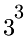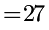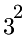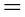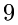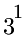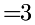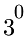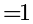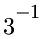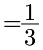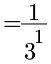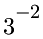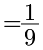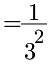### Directions:

You need to identify the numerical expression that is equivalent to the given fraction. Strive to be able to accomplish the problems with ease and then strive to increase your speed. Good luck and enjoy the challenge!

...